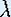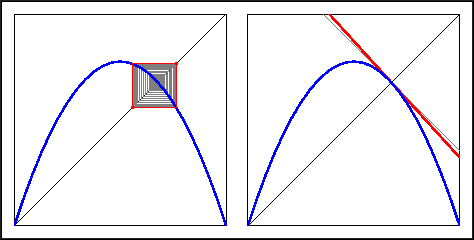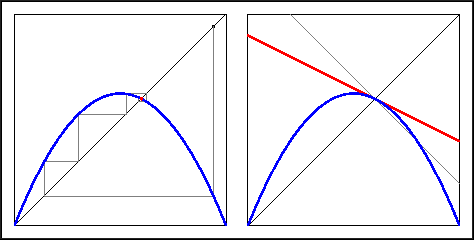Up: Linear and Nonlinear Behavior

# One-Dimensional Dynamical Systems

## Part 4: Linear and Nonlinear Behavior

#### Hartman and Grobman

Locally near a point x, a differential function can be approximated by its derivative at x. That is, the graph of the function locally near x is almost equal to a straight line with a slope equal to the derivative at x. Hence, the derivative Df(x) at a specific point x can be interpreted as a (linear) map, that has this straight line as its graph. This map is called the derivative map. However, if the functions are almost the same near x, what about the behavior of the two dynamical systems?

Note that the theorem is only true on a small neighborhood of the fixed point p. In particular if other fixed points or periodic points are close to p, this neighborhood is very small! You can now find a relationship between the slope of the graph at the fixed point and whether the fixed point is attracting, repelling, or neutral.The fixed point other than 0 is attracting for= 1.5.

Recall your investigations in the Warm Up Questions. In the figure above (right) the graph of the derivative map at the fixed point is drawn in read. Also drawn are the two special lines with slope 1 and -1. The figure clearly shows that the derivative at the fixed point lies in between -1 and 1, so that the fixed point is attracting.The fixed point other than 0 is repelling for= 3.1.

For= 3.1 the graph of the derivative map no longer has a slope between -1 and 1. Hence, the fixed point is now repelling.

• What are the slopes of the graph of the Logistic map at the fixed points for= 1.5 and 3.1?The fixed point other than 0 is attracting for= 2.5.

• Compare the figure for= 2.5 with the figure for= 1.5. Notice the difference in behavior of the graphical iterations. Why do you think this difference is there? (Hint: look at the slope of the graph at the attracting fixed point.)

• Recall that in order to find period-2 points, you graphed the second iterate of the map. Can you find a relationship between the slope of the graph of the second iterate of the function and whether the period-2 points are attracting, repelling, or neutral?

• Describe geometrically how your relationship might generalize to period-n points.

Note: The procedure you found only works for periodic points with small periods. Although it is nice to have the condition above for whether a period-n point is attracting, it becomes computationally difficult to accurately graph the n-th iterate of a map as n becomes large.

Up: Linear and Nonlinear BehaviorThe Geometry Center Home Page

Written by Hinke Osinga